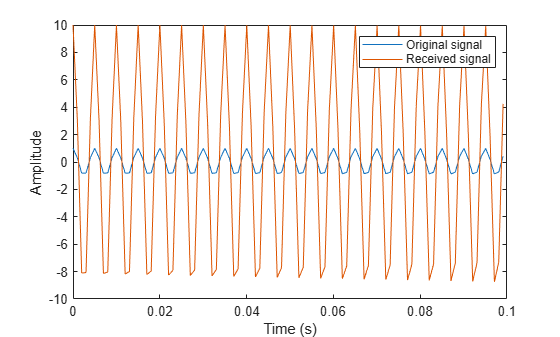# step

Package: phased

## Syntax

```Y = step(H,X) Y = step(H,X,EN_RX) Y = step(H,X,PHNOISE) Y = step(H,X,EN_RX,PHNOISE) ```

## Description

Note

Starting in R2016b, instead of using the `step` method to perform the operation defined by the System object™, you can call the object with arguments, as if it were a function. For example, ```y = step(obj,x)``` and `y = obj(x)` perform equivalent operations.

`Y = step(H,X)` applies the receiver gain and the receiver noise to the input signal, `X`, and returns the resulting output signal, `Y`.

`Y = step(H,X,EN_RX)` uses input `EN_RX` as the enabling signal when the `EnableInputPort` property is set to `true`.

`Y = step(H,X,PHNOISE)` uses input `PHNOISE` as the phase noise for each sample in `X` when the `PhaseNoiseInputPort` is set to `true`. The phase noise is the same for all channels in `X`. The elements in `PHNOISE` represent the random phases the transmitter adds to the transmitted pulses. The receiver preamp object removes these random phases from all received samples returned within corresponding pulse intervals. Such setup is often referred to as coherent on receive.

`Y = step(H,X,EN_RX,PHNOISE)` combines all input arguments. This syntax is available when you configure `H` so that `H.EnableInputPort` is `true` and `H.PhaseNoiseInputPort` is `true`.

Note

The object performs an initialization the first time the object is executed. This initialization locks nontunable properties and input specifications, such as dimensions, complexity, and data type of the input data. If you change a nontunable property or an input specification, the System object issues an error. To change nontunable properties or inputs, you must first call the `release` method to unlock the object.

## Input Arguments

 `H` Receiver object. `X` Input signalThe size of the first dimension of the input matrix can vary to simulate a changing signal length. A size change can occur, for example, in the case of a pulse waveform with variable pulse repetition frequency. `EN_RX` Enabling signal, specified as a column vector whose length equals the number of rows in `X`. The data type of `EN_RN` is `double` or `logical`. Every element of `EN_RX` that equals `0` or `false` indicates that the receiver is turned off, and no input signal passes through the receiver. Every element of `EN_RX` that is nonzero or `true` indicates that the receiver is turned on, and the input passes through. `PHNOISE` Phase noise for each sample in `X`, specified as a column vector whose length equals the number of rows in `X`. You can obtain `PHNOISE` as an optional output argument from the `step` method of `phased.Transmitter`.

## Output Arguments

 `Y` Output signal. `Y` has the same dimensions as `X`.

## Examples

expand all

This example shows how to construct a `phased.ReceiverPreamp` System object™ with a noise figure of 5 dB and a bandwidth of 1 MHz. Then use the object to amplify the signal.

Construct the Receiver Preamp System object.

`receiver = phased.ReceiverPreamp('NoiseFigure',5,'SampleRate',1e6);`

Create the signal.

```Fs = 1e3; t = linspace(0,1,1e3); x = cos(2*pi*200*t)';```

Use the `step` method to amplify the signal and then plot the first 100 samples.

```y = receiver(x); idx = [1:100]; plot(t(idx),x(idx),t(idx),real(y(idx))) xlabel('Time (s)') ylabel('Amplitude') legend('Original signal','Received signal')```